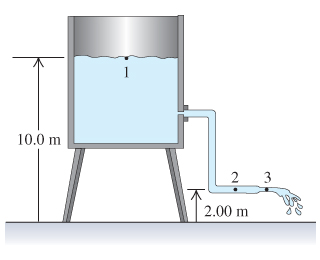# Problem: Water flows steadily from an open tank as shown in the figure. (Figure 1) The elevation of point 1 is 10.0m, and the elevation of points 2 and 3 is 2.00 m. The cross-sectional area at point 2 is 4.80x102m; at point 3, where the water is discharged, it is 1.60x102m. The cross-sectional area of the tank is very large compared with the cross-sectional area of the pipe. Part A Assuming that Bernoulli's equation applies, compute the volume of water ΔV that flows across the exit of the pipe in 1.00 s. In other words, find the discharge rate ΔV/Δt. Express your answer numerically in cubic meters per second.

###### FREE Expert Solution

We are told to use Bernoulli's equation.

Bernoulli's equation:

$\overline{){{\mathbf{P}}}_{{\mathbf{1}}}{\mathbf{+}}\frac{\mathbf{1}}{\mathbf{2}}{\mathbf{\rho }}{{{\mathbf{v}}}_{{\mathbf{1}}}}^{{\mathbf{2}}}{\mathbf{+}}{{\mathbf{h}}}_{{\mathbf{1}}}{\mathbf{\rho }}{\mathbf{g}}{\mathbf{=}}{{\mathbf{P}}}_{{\mathbf{2}}}{\mathbf{+}}\frac{\mathbf{1}}{\mathbf{2}}{\mathbf{\rho }}{{\mathbf{v}}_{\mathbf{2}}}^{{\mathbf{2}}}{\mathbf{+}}{{\mathbf{h}}}_{{\mathbf{2}}}{\mathbf{\rho }}{\mathbf{g}}}$

Flow rate:

$\overline{){\mathbf{Q}}{\mathbf{=}}{\mathbf{A}}{\mathbf{v}}}$

96% (176 ratings)###### Problem DetailsWater flows steadily from an open tank as shown in the figure. (Figure 1) The elevation of point 1 is 10.0m, and the elevation of points 2 and 3 is 2.00 m. The cross-sectional area at point 2 is 4.80x102m; at point 3, where the water is discharged, it is 1.60x102m. The cross-sectional area of the tank is very large compared with the cross-sectional area of the pipe.

Part A Assuming that Bernoulli's equation applies, compute the volume of water ΔV that flows across the exit of the pipe in 1.00 s. In other words, find the discharge rate ΔV/Δt. Express your answer numerically in cubic meters per second.